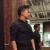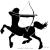## 面试题 类似约瑟夫环

0```import java.util.ArrayList;
import java.util.List;
import java.util.ListIterator;

public class Main {

public static void main(String[] args) {
final int n = 15;
final int flag = 3;

List<Integer> mans = new ArrayList<Integer>();
for (int i = 0; i < n; i++) {
mans.add(i + 1);
}
System.out.println("初始化编号为" + mans + "的" + mans.size() + "个人围成一圈");

ListIterator<Integer> iter = null;
int k = 1;
do {
iter = mans.listIterator();
while (iter.hasNext()) {
int i = iter.next();
if (k++ % flag == 0) {
System.out.println("编号" + i + "的人退出圈子");
iter.remove();
k = 1;
}
}
} while (mans.size() > 1);

System.out.println("剩下编号为" + mans + "的" + mans.size() + "个人");
}

}```

0```def joseph(n: Int, k:Int, end: Int) = {
var r = 0
for(i <- 1 to n) {
r = (r + k) % i
}
end - r
}
assert(joseph(15, 3, 15) == 11)
assert(joseph(16, 3, 15) == 8)
assert(joseph(17, 3, 15) == 5)```